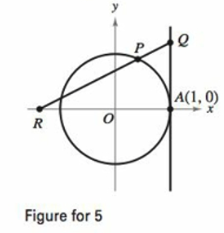Chapter 8, Problem 5PS

Chapter
Section
Textbook Problem

# Length The line x = 1 is tangent to the unit circle at A. The length of segment QA equals the length of the circular are PA (see figure). Show that the length of segment OR approaches 2 as P approaches A.To determine

To prove: That the length of segment OR tends to 2 as P approaches to A, for the provided circle which has a tangent line x=1 at A and the length of the segment QA equals the length of the circular arc PA.

Explanation

Given:

The provided tangent line is x=1 to the unit circle at A and refers to the figure provided in the question.

Formula used:

The L’Hopitals rule is,

limxND=00

Then,

limxND=limxdNdD

Proof:

Consider the provided figure,

Now, draw a perpendicular line from point P on the OA line and connect two-point O and P as shown below,

As, P approaches to A the angle would be very less,

Now from the triangle POB,

sinθ=PBOPPB

Therefore,

cosθ=OBOPOB

Because the angle θ is very small so that,

AQ=AP=θ

Therefore, the BR is,

BR=OR+OB=OR+cosθ

From the figure the triangle QAR and BPR are similar,

So that,

ARAQ=BRBPOR+1θ=OR+cosθsinθ

Therefore,

sinθ(OR)+sinθ=(OR)θ+θcosθΟR=θcosθsinθ

### Still sussing out bartleby?

Check out a sample textbook solution.

See a sample solution

#### The Solution to Your Study Problems

Bartleby provides explanations to thousands of textbook problems written by our experts, many with advanced degrees!

Get Started

## Additional Math Solutions

#### Find more solutions based on key concepts